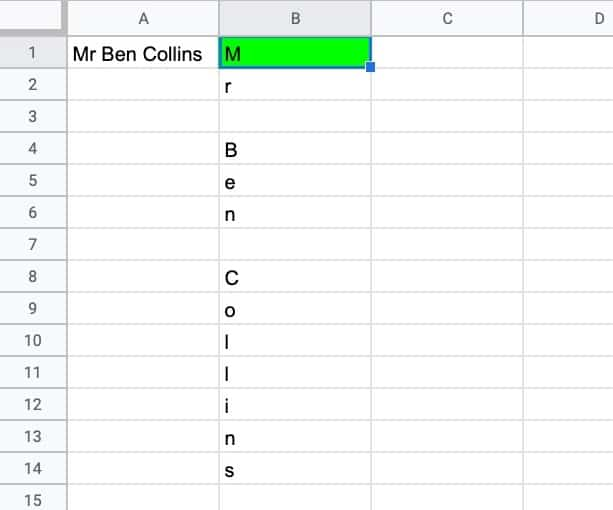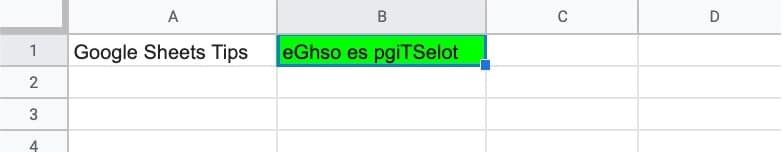# Formula Challenge #6: Split A String Into Characters And Recombine In Random Order

This Formula Challenge originally appeared as Tip #194 of my weekly Google Sheets Tips newsletter, on 7 March 2022.

Congratulations to everyone who took part and well done to the 97 people who submitted a solution!

Special mention to Kieran D., Louise A., Martin H., Jelle G., Karl S., Earl N., Doug S., JP C., Alan B., and others for their ingenious solutions. I’ve shared the best below.

Sign up here so you don’t miss out on future Formula Challenges:

## The Challenge Part I: Split A String Into Characters

Question: can you create a single formula to split a string into characters so that each character is in its own cell?

i.e. can you create a single formula in cell B1 that creates the output shown in this example:### Solution 1: Using MID and SEQUENCE

Assuming the string is in cell A1, then this formula outputs the characters in a vertical orientation (column):

`=ArrayFormula(MID(A1,SEQUENCE(LEN(A1)),1))`

To change to a horizontal orientation (row), modify the formula to:

`=ArrayFormula(MID(A1,SEQUENCE(1,LEN(A1)),1))`

Complex formulas are best understood by working through layer-by-layer, starting from the inside, using the onion framework.

Applying that here, we begin with a LEN function to calculate the length of the string in A1.

The SEQUENCE function creates a numbered list 1, 2, 3, etc. up to the value corresponding to the number of characters in the original string.

Next the MID function splits the string into single characters and the array formula applies it to each character.

### Solution 2: Using REGEXREPLACE and SPLIT

`=SPLIT(REGEXREPLACE(A1,"","✂️"),"✂️")`

This succinct and beautiful formula uses the REGEXREPLACE formula to insert a character between each character in the original string.

The SPLIT function is then used to break this new string apart based on the inserted character “✂️”.

As it’s written above, this formula outputs the characters in a horizontal orientation. To change to a vertical orientation, wrap it with a TRANSPOSE function.

### Solution 3: Using REGEXEXTRACT and REPT

`=REGEXEXTRACT(A1, REPT("(.)", LEN(A1)))`

This is another clever formula to split a string into characters.

It begins with the REPT function and LEN function to create a string like this (.)(.)(.)…etc., where the number of bracket groups corresponds to the number of characters in the original string.

This feeds into the REGEXEXTRACT formula which extracts each bracket as a single character group in its own cell. Very cool!

Again, this output is a horizontal output, so wrap it with a TRANSPOSE function to switch to a vertical orientation.

### Solution 4: Use REGEXEXTRACT and REGEXREPLACE

`=REGEXEXTRACT(A1,REGEXREPLACE(A1,"(.)","(\$1)"))`

Another exceedingly clever REGEX formula solution!

The REGEXREPLACE adds brackets around each character in the string, e.g. “Google” becomes “(G)(o)(o)(g)(l)(e)”

Next, REGEXEXTRACT extracts each bracket group into its own cell.

As with earlier solutions, TRANSPOSE can be used to convert to a vertical orientation.

## The Challenge Part II: Recombine In Random Order

Question: can you create a single formula to randomize the characters in a string?

i.e. can you create a single formula in cell B1 to create a copy of the string with the letters in random order:The key to this question was to use the solution from part 1 inside a SORT function and use a random array to randomize the order.

Here’s the full formula to randomize the characters of a string in cell A1:

`=JOIN("",SORT(MID(A1,SEQUENCE(LEN(A1)),1),RANDARRAY(LEN(A1)),1))`

The RANDARRAY function creates a random array with a length matching the length of the string in cell A1, calculated by the LEN function.

This is then fed into the SORT function as the sorting column. The characters are passed to the SORT function as the range to sort.

Finally, the list of randomized characters is joined together with the JOIN function.

Note how it doesn’t require the array formula designation because it all happens within the SORT function, which can already deal with arrays.

## 2 thoughts on “Formula Challenge #6: Split A String Into Characters And Recombine In Random Order”

1.Michel says:

Hi,
Just heard of this challenge from colleagues last week but never heard of SEQUENCE before. So I made it with formulas that are more usual for me.
=ArrayFormula(MID(\$A\$1;ROW(B1:indirect(“B”&LEN(A1)));1))
It works as well.

2.A says: